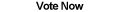Home Articles Components Code Snippets Links# C# Dominant Color in Image

## Dominant Image Color

A simple algorithm in C# can extract the dominant color in an image. The .NET Framework does most work the work in reading the image.

The dominant color algorithm is useful for determening the overall tone of an image. Keep in mind that his algorithm is very simple and there are probably more complex and thus better color algorithms out there.

## C# Extract Dominant Color

The dominant color is extracted by going through every pixel in the image (using .NET GDI+). For each pixel, we keep track of three values: the red value, the green value, and the blue value (RGB). In this article we won't worry about the alpha value (transperancy).

So as we go through each pixel, we keep a tally of all the red values, all the green values, and all the blue values. After going through the entire image, we can now tell which of the three values showed up the most, thus the domintant color.

To calculate the average color we need a fourth value, which is the amount of pixels in the entire image (width * height). By dividing each of the total red, blue, and green values with the total amount of pixels, we calculate the average color of the image.

## Shortcomings

That's it! Pretty simple right? But notice that the algorithm does not really calculate a true dominant color, it is more of an average color. This is because the colors of all pixels in the image are treated with the same "weight". However for a simple algorithm it gets the work done.

Unfortunately part of .NET GDI+ is that it is slow at reading pixels. Luckily there are ways so processes images faster. And of course, you want to be able to load images in the first place.

Back to C# Article List

Other C# Articles